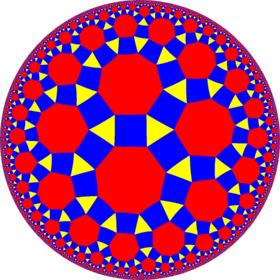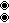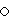# Rhombitrioctagonal tiling

Rhombitrioctagonal tilingPoincaré disk model of the hyperbolic plane
TypeHyperbolic uniform tiling
Vertex configuration3.4.8.4
Schläfli symbolrr{8,3} ors2{3,8}
Wythoff symbol3 | 8 2
Coxeter diagramorSymmetry group[8,3], (*832)
[8,3+], (3*4)
DualDeltoidal trioctagonal tiling
PropertiesVertex-transitive

In geometry, the rhombitrioctagonal tiling is a semiregular tiling of the hyperbolic plane. At each vertex of the tiling there is one triangle and one octagon, alternating between two squares. The tiling has Schläfli symbol rr{8,3}. It can be seen as constructed as a rectified trioctagonal tiling, r{8,3}, as well as an expanded octagonal tiling or expanded order-8 triangular tiling.

## Symmetry

This tiling has [8,3], (*832) symmetry. There is only one uniform coloring.

Similar to the Euclidean rhombitrihexagonal tiling, by edge-coloring there is a half symmetry form (3*4) orbifold notation. The octagons can be considered as truncated squares, t{4} with two types of edges. It has Coxeter diagram, Schläfli symbol s2{3,8}. The squares can be distorted into isosceles trapezoids. In the limit, where the rectangles degenerate into edges, an order-8 triangular tiling results, constructed as an snub tritetratrigonal tiling,.

From a Wythoff construction there are ten hyperbolic uniform tilings that can be based from the regular octagonal tiling.

Drawing the tiles colored as red on the original faces, yellow at the original vertices, and blue along the original edges, there are 8 forms.

### Symmetry mutations

This tiling is topologically related as a part of sequence of cantellated polyhedra with vertex figure (3.4.n.4), and continues as tilings of the hyperbolic plane. These vertex-transitive figures have (*n32) reflectional symmetry.Wikimedia Commons has media related to Uniform tiling 3-4-8-4.